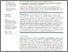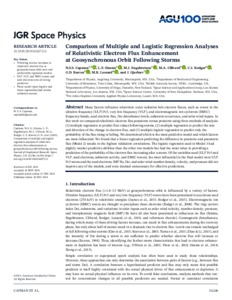nerc.ac.uk

# Comparison of multiple and logistic regression analyses of relativistic electron flux enhancement at geosynchronous orbit following storms

Capman, N.S.S.; Simms, L.E.; Engebretson, M.J.; ; Rodger, C.J.; Reeves, G.D.; Lessard, M.R.; Gjerloev, J.. 2019 Comparison of multiple and logistic regression analyses of relativistic electron flux enhancement at geosynchronous orbit following storms. Journal of Geophysical Research: Space Physics, 124 (12). 10246-10256. https://doi.org/10.1029/2019JA027132Preview
Text
Capman_et_al-2019-Journal_of_Geophysical_Research__Space_Physics.pdf - Published Version

## Abstract/Summary

Many factors influence relativistic outer radiation belt electron fluxes, such as waves in the ultra low frequency (ULF) Pc5, very low frequency (VLF), and electromagnetic ion cyclotron (EMIC) frequency bands, seed electron flux, Dst disturbance levels, substorm occurrence, and solar wind inputs. In this work we compared relativistic electron flux post storm vs. pre‐storm using three methods of analysis: 1) multiple regression to predict flux values following storms, 2) multiple regression to predict the size and direction of the change in electron flux, and 3) multiple logistic regression to predict only the probability of the flux rising or falling. We determined which is the most predictive model, and which factors are most influential. We found that a linear regression predicting the difference in pre‐storm and post storm flux (Model 2) results in the highest validation correlations. The logistic regression used in Model 3 had slightly weaker predictive abilities than the other two models, but had most value in providing a prediction of the probability of the electron flux increasing after a storm. Of the variables used (ULF Pc5 and VLF waves, seed electrons, substorm activity, and EMIC waves), the most influential in the final model were ULF Pc5 waves and the seed electrons. IMF Bz, Dst, and solar wind number density, velocity, and pressure did not improve any of the models, and were deemed unnecessary for effective predictions.

Item Type: Publication - Article https://doi.org/10.1029/2019JA027132 21699380 multiple regression, logistic regression, ULF waves, VLF waves, EMIC waves 20 Dec 2019 10:57 +0 (UTC) https://nora.nerc.ac.uk/id/eprint/526327View Item